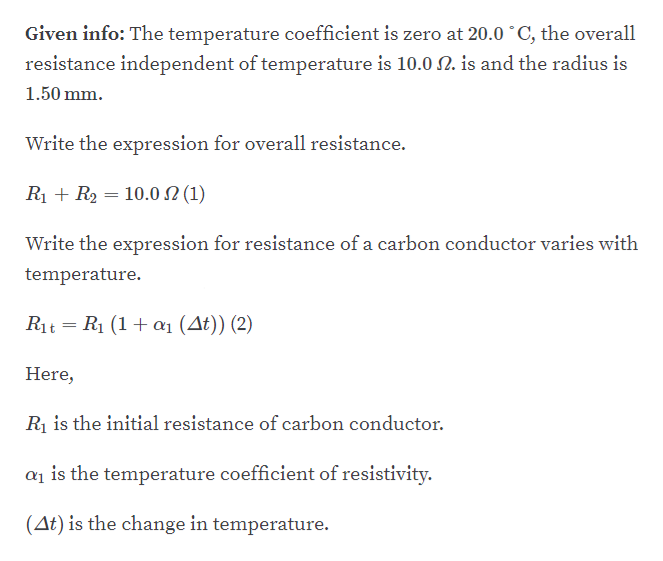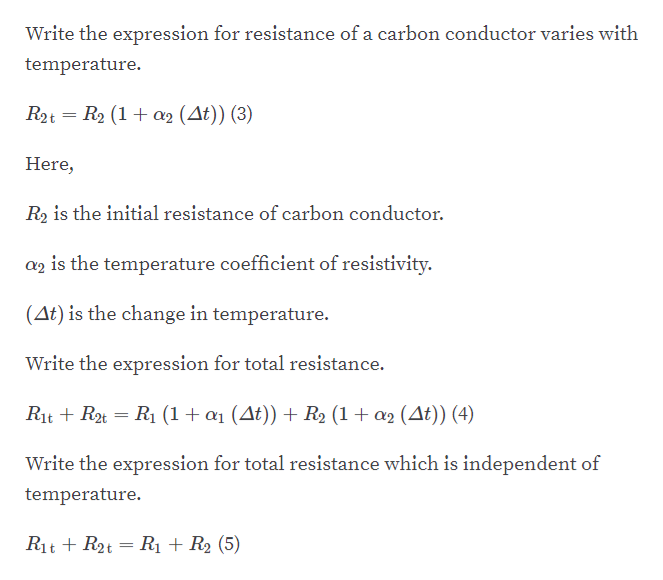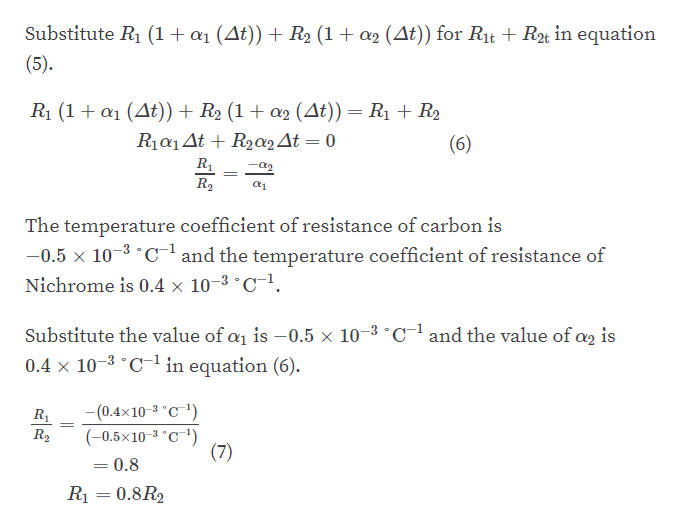Question
10 views

An engineer needs a resistor with a zero overall temperature
coefficient of resistance at 20.0°C. She designs a pair of circular
cylinders, one of carbon and one of Nichrome as shown in
Figure P17.32. The device must have an overall resistance of
R1 + R2 = 10.0 Ω independent of temperature and a uniform
radius of r = 1.50 mm. Ignore thermal expansion of the cylinders
and assume both are always at the same temperature.
(a) Can she meet the design goal with this method? (b) If so,
state what you can determine about the lengths L1 and L2 of
each segment. If not, explain.

check_circle

Step 1

part A:help_outlineImage TranscriptioncloseGiven info: The temperature coefficient is zero at 20.0 °C, the overall resistance independent of temperature is 10.0 N. is and the radius is 1.50 mm. Write the expression for overall resistance. R1 + R2 = 10.0 N (1) Write the expression for resistance of a carbon conductor varies with temperature. R1t = R1 (1+ a1 (At)) (2) Here, Rị is the initial resistance of carbon conductor. ai is the temperature coefficient of resistivity. (At) is the change in temperature. fullscreen
Step 2help_outlineImage TranscriptioncloseWrite the expression for resistance of a carbon conductor varies with temperature. R2t = R2 (1+ a2 (At)) (3) Here, R2 is the initial resistance of carbon conductor. az is the temperature coefficient of resistivity. (At) is the change in temperature. Write the expression for total resistance. Rit + R2t = R1 (1+ a1 (At)) + R2 (1+ a2 (At)) (4) Write the expression for total resistance which is independent of temperature. Rit + R2t = R1 + R2 (5) fullscreen
Step 3help_outlineImage TranscriptioncloseSubstitute R1 (1+a1 (At)) + R2 (1+ a2 (At)) for Rt + Rt in equation (5). R1 (1+ a1 (At)) + R2 (1 + a2 (At)) = R1 + R2 R1a1At + R2a2At = 0 (6) R1 -a2 R2 The temperature coefficient of resistance of carbon is -0.5 x 10-3 °C-l and the temperature coefficient of resistance of Nichrome is 0.4 × 10-3 °C. Substitute the value of a is -0.5 × 10–3 °Cland the value of ag is 0.4 × 10-3 °C-1 in equation (6). -(0.4×10-3 °C¯1) R1 (-0.5×10-3 °C¯') (7) R2 0.8 R1 = 0.8R2 fullscreen

### Want to see the full answer?

See Solution

#### Want to see this answer and more?

Solutions are written by subject experts who are available 24/7. Questions are typically answered within 1 hour.*

See Solution
*Response times may vary by subject and question.
Tagged in